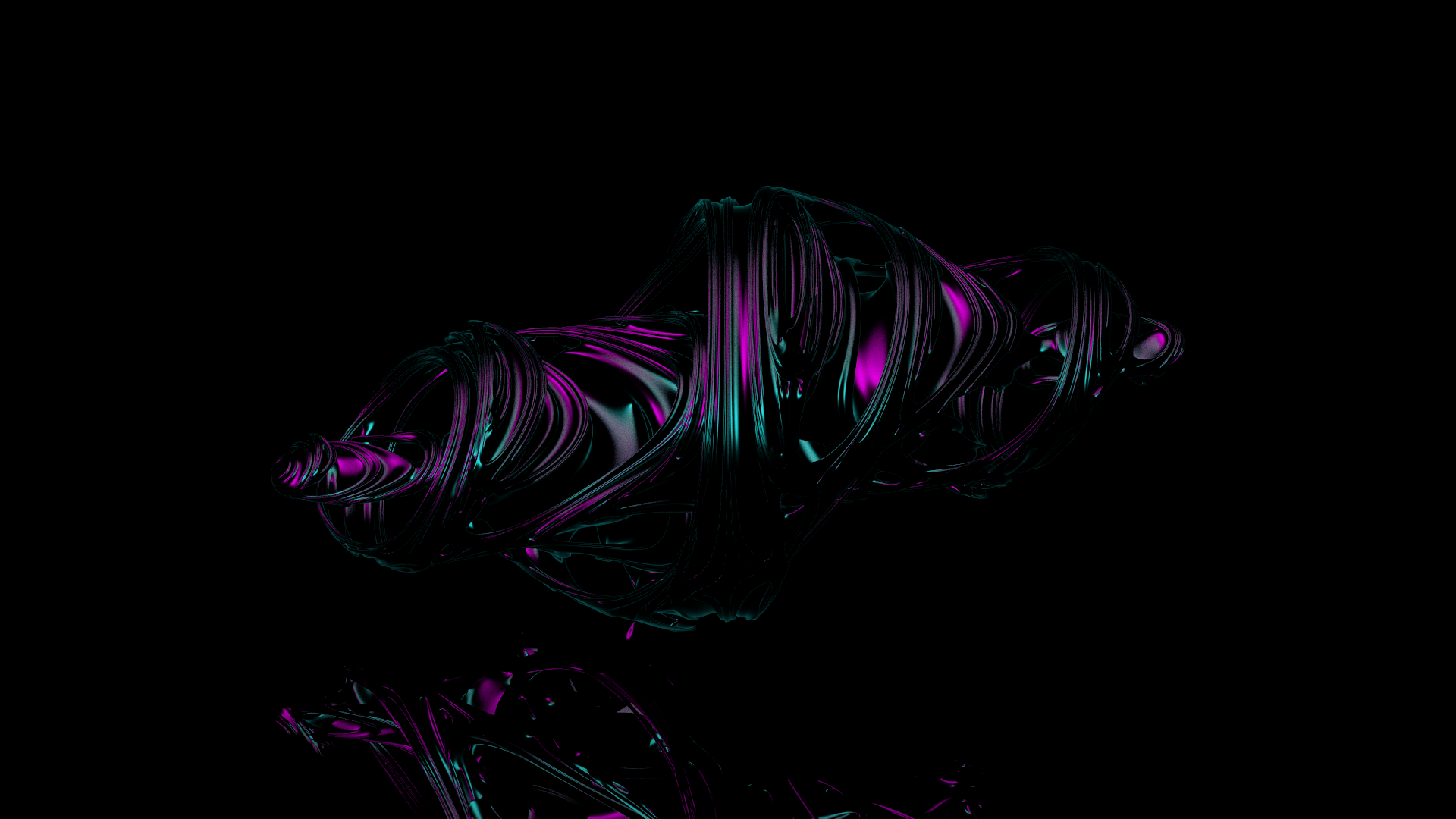## Short descriptionWhat you see in the image is a render of the complex Julia set we all know and love extended to the 4th dimension and projected back into 3D space. I was always fascinated of Julia sets and fractals and wanted to create a visualization myself. Originally, we wanted to go for a scene similar to scenes in this video.

We rendered the image raymarching the signed distance function of the Julia set. That means that - instead of computing the intersection of ray with the set - we start from the origin and take steps in the direction of the ray until we reach a point that is contained in the set. We are using a distance estimator to adapt the step size and speed up the intersection computation. The normal at the intersection point is computed by a simple finite difference method. For more math read this wonderful article by Inigo Quilez.

The scene is just a simple plane with a mirror material, two area lights (one pink and one cyan) and the Phong shaded fractal solid. The final render was done with 300 times supersampling and took 19 minutes and 55 seconds on 8 cores.

The animation in the background has 30 frames and only uses about 40 times supersampling since we did not have time to render 30 frames at full 300 times supersampling.

## Implementation

Since the renderer takes quite a long time to render a 1920x1080 image, we decided to parallelize it since raytracing is an "embarrasingly parallel" problem. Fortunately, this is easily done in C++ 2017 standard by using execution policies:

```std::for_each(
std::execution::par,
pixels.begin(), pixels.end(),
[&](const std::pair<int, int> &px) {
img(px.first, px.second) = renderPixel(px.first, px.second);
});
```

We implemented the possibility to render both square and cubic Julia sets, but our final render displays a square Julia set.

```float squareSdf(const Point &p, const Float4 &c) {
Float4 z{p.x, p.y, p.z, 0};

float md2 = 1.0;
float mz2 = qLengthSqr(z);

for (int i = 0; i < NUM_ITER; ++i) {
md2 *= 4.0 * mz2;
z = qSqr(z) + c;

mz2 = qLengthSqr(z);
if (mz2 > 256)
break;
}

float d = 0.25 * std::log(mz2) * std::sqrt(mz2 / md2);

return d;
}
```

The intersection was done by iteratively taking small steps along the direction of the ray, calculating the signed distance function and breaking if the distance is small enough. Once we have the hit point on the surface of the Julia set, we calculate the normal by means of a simple finite difference method:

```Vector Fractal::computeNormal(const Point &p) const {
float ex = 0.5773 * 0.00025;
float ey = -0.5773 * 0.00025;

Vector exyy = Vector(ex, ey, ey);
Vector eyyx = Vector(ey, ey, ex);
Vector eyxy = Vector(ey, ex, ey);
Vector exxx = Vector(ex, ex, ex);

return (exyy * sdf(p + exyy)
+ eyyx * sdf(p + eyyx)
+ eyxy * sdf(p + eyxy)
+ exxx * sdf(p + exxx)).normalize();
}
```

The generated image is located in the build folder named "fractal.png"